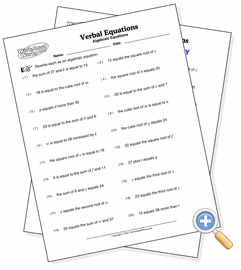# Verbal Equations

## Algebraic EquationsRead and understand algebraic verbal equations

This worksheet describes algebraic equations verbally and asks the student to write them in algebraic form. The challenge is to understand the terminology used, such as “less than,” “quotient of,” and “increased by.”